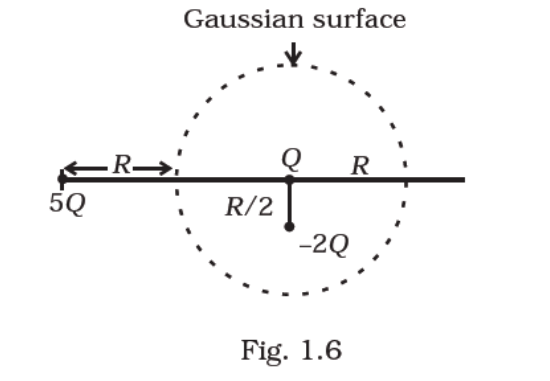# Refer to the arrangement of charges in`
Question:

Refer to the arrangement of charges in Fig. 1.6 and a Gaussian surface of radius R with Q at the centre. Then

(a) total flux through the surface of the sphere is ‒Qo

(b) field on the surface of the sphere is ‒Q/(4πεoR2)

(c) flux through the surface of sphere due to 5Q is zero

(d) field on the surface of sphere due to –2Q is same everywhereSolution:

Gauss' law states that total electric flux of an enclosed surface is given by qo. Here, is the net charge enclosed by the Gaussian surface.

From the figure,

Net charge inside the surface is = Q ‒ 2= ‒ Q.

Net flux through the surface of the sphere = ‒ Qo

Here, charge 5Q lies outside the Gaussian surface, so it will not make no contribution to electric flux through the given surface.